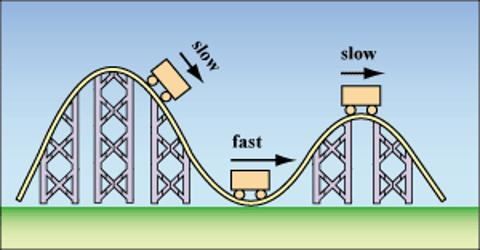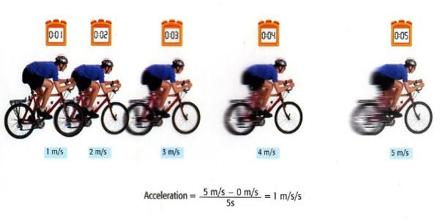# Acceleration: Definition and Calculation

Acceleration is defined as the rate of change of velocity. If you’re not changing your speed and you’re not changing your direction, then you simply cannot be accelerating—no matter how fast you’re going.

Suppose a motor-cycle was running with a velocity of 20 meter/second. As a result of pressing on the accelerator by the driver the motor-cycle was going with more velocity.

Explanation and Calculation

Suppose the velocity of the motor-cycle was increasing at the rate of 5 meter/second. The primary or initial velocity of the motor-cycle was 20 meter/second.

• After 1 second the velocity increased to 25 meter/second.
• After 2 second the velocity increased to 30 meter/second.
• After 3 second the velocity increased to 35 meter/second.
• After 4 second the velocity increased to 40 meter/second.
• After 5 second the velocity increased to 45 meter/second.The amount of velocity increasing per second is the acceleration. Here the velocity increasing per second is 5 meter/second. Hence, acceleration per second is 5 meter/second, which can be written as 5 meter second. If the total increase of velocity and total time are known, the acceleration can be easily found out.

Here, the initial velocity was 20 meter second and final velocity was 45 meter second. Here, the initial velocity was 20 meter second and final velocity was 45 meter second.

Hence, Total increase in velocity is, [final velocity – initial velocity] = (45 – 20) meter/second = 25 meter/second. Total time taken is 5 seconds.

so, Acceleration = [Total increase in velocity/Total time] = (25 m/s)/5s = 5 m/s-2

Hence, acceleration is the rate of increase in velocity with time and increase in velocity per second.